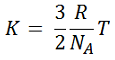# Problem: Calculate the kinetic energy of F2, Cl2, and Br2 at 302 K .

🤓 Based on our data, we think this question is relevant for Professor Dixon's class at UCF.

###### FREE Expert Solution

The average kinetic energy of each gas can be calculated using the equation below:where:

K= Average kinetic energy
R= gas constant (8.314 J/mol-K)
NA = Avogadro's number (6.022x1023 mol-1)
T = Temperature in Kelvin###### Problem Details

Calculate the kinetic energy of F2, Cl2, and Br2 at 302 K .